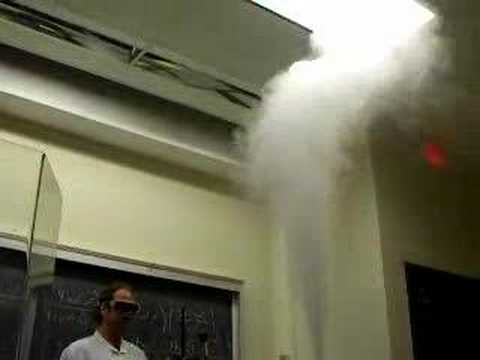# Hydrogen peroxide and manganese (ii) sulfate yields..?

In a lab experiment, we combined H2O2 and MnSO4. We put a recently burnt match over the reaction and the matchstick glowed for a moment. That could mean that oxygen evolved from the reaction.

What then is the chemical equation for the reaction? I tried creating a chemical equation and came up with:

H2O2 + MnSO4 --> Mn(HSO4)2 + O2.

But some claim it to yield H2SO4 and MnO2. The problem with this is that sulfuric acid is liquid, while manganese dioxide is a gas. There is no oxygen which may cause the glow.

Relevance

MnSO4 reacts with H2O2 to give H2SO4 and MnO2 itself. but in presence of acidic medium, MnO2 reacts with excess of H2O2 to give oxygen

MnO2 + H2SO4 + H2O2 -----> MnSO4 + H20 + O2

so when you conducted the experiment in the lab, excess of H2O2 present must have reacted with the product formed that is MnO2 hence liberating oxygen

and hence the glowing of the matchstick

• Oh please!!! MnO2 is NOT A GAS.

Hydrogen peroxide can be either an oxidizing agent or a reducing agent, depending upon what it is reacting with.

For H2O2 to produce O2 then the oxygen in H2O2 is oxidized and manganese would need to be reduced. But guess what. Mn is already in its lowest oxidation state and can't be reduced (unless to Mn metal).

Therefore something else gets to be reduced. How about the S in sulfate ion? It could get reduced to SO2 gas or sulfite ion.

H2O2(aq) + SO4= --> O2 + SO3= + H2O

I'm afraid that what you suggested won't work.

H2O2 + MnSO4 --> Mn(HSO4)2 + O2.

You only have oxygen being oxidized, but nothing is being reduced.

Monica -- This makes no sense. Why would H2O2 react with Mn2+ to make MnO2 and then MnO2 react with H2O2 to go back to Mn2+?

• Anonymous

The reaction occurs in 2 steps:

1) MnSO4 (aq) + H2O2 (aq) --> MnO2 (s) + H2SO4 (aq) (see below for net ionic equation and half-reactions)

2) 2H2O2 (aq) --> 2H2O (l) + O2 (g)

The second reaction is very efficiently catalyzed by solid MnO2 (very well known, see source and this youtube video: http://www.youtube.com/watch?v=u7nrQ6dNsXM), so once you generate just a bit of MnO2 in the first reaction, the second one kicks in and destroys the rest of the H2O2. The second reaction generates the oxygen that causes the matchstick to glow.

Net ionic equation for the first reaction:

Mn^2+ (aq) + H2O2 (aq) --> MnO2(aq) + 2H+ (aq)

The half reactions for the first reaction are:

Oxidation: Mn^2+ (aq) + 2H2O (l) --> MnO2 (s) + 4H+ (aq) +2e-

Reduction: H2O2 (aq) + 2H+ (aq) + 2e- --> 2H2O (l)